JEE  >  Thermodynamics MCQ - 4 (Advanced)

# Thermodynamics MCQ - 4 (Advanced)

Test Description

## 10 Questions MCQ Test Chemistry for JEE Advanced | Thermodynamics MCQ - 4 (Advanced)

Thermodynamics MCQ - 4 (Advanced) for JEE 2022 is part of Chemistry for JEE Advanced preparation. The Thermodynamics MCQ - 4 (Advanced) questions and answers have been prepared according to the JEE exam syllabus.The Thermodynamics MCQ - 4 (Advanced) MCQs are made for JEE 2022 Exam. Find important definitions, questions, notes, meanings, examples, exercises, MCQs and online tests for Thermodynamics MCQ - 4 (Advanced) below.
Solutions of Thermodynamics MCQ - 4 (Advanced) questions in English are available as part of our Chemistry for JEE Advanced for JEE & Thermodynamics MCQ - 4 (Advanced) solutions in Hindi for Chemistry for JEE Advanced course. Download more important topics, notes, lectures and mock test series for JEE Exam by signing up for free. Attempt Thermodynamics MCQ - 4 (Advanced) | 10 questions in 40 minutes | Mock test for JEE preparation | Free important questions MCQ to study Chemistry for JEE Advanced for JEE Exam | Download free PDF with solutions
 1 Crore+ students have signed up on EduRev. Have you?
Thermodynamics MCQ - 4 (Advanced) - Question 1

### For a reversible reaction at constant temperature and at constant pressure the equilibrium composition of reaction mixture corresponds to the lowest point on Gibbis energy Vs progress of reaction diagrams as shown. At equilibrium Gibbs energy of reaction is equal to Zero.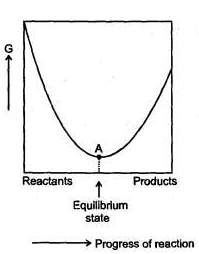Q. The value of log10 keg is equal to Kg is the equilibrium constant]

Thermodynamics MCQ - 4 (Advanced) - Question 2

### For a reversible reaction at constant temperature and at constant pressure the equilibrium composition of reaction mixture corresponds to the lowest point on Gibbis energy Vs progress of reaction diagrams as shown. At equilibrium Gibbs energy of reaction is equal to Zero.Q. Which diagram represents the large value of equilibrium constant for the reversible reaction

Thermodynamics MCQ - 4 (Advanced) - Question 3

### For a reversible reaction at constant temperature and at constant pressure the equilibrium composition of reaction mixture corresponds to the lowest point on Gibbis energy Vs progress of reaction diagrams as shown. At equilibrium Gibbs energy of reaction is equal to Zero.For a reaction M2 O(s)→ 2M(S) →2M(s) + 1/2O2(g) ΔH = 30 KJ/mol and ΔS = 0.07 KJ/mol /K at latm. The reaction would not be spontaneous at  temperatures

Thermodynamics MCQ - 4 (Advanced) - Question 4

The contributions of both heat (enthalpy) and randomness (entropy) shall be considered to the overall spontaneity of a process. When deciding about the spontaneity of a chemical reaction or other process, we define a quantity called the Gibb's energy change (ΔG).

ΔG = ΔH – TΔS

where,        ΔH = Enthalpy change ; ΔS = Entropy change;

T = Temperature in kelvin.

If ΔG < 0, Process is spontaneous ; ΔG = 0, Process is at equilibrium, AG > 0, Process is non-spontaneous.

Q.

For the change

H20 (s, 273 K, 2 atm) → H20 (l, 273K, 2 atom),

choose the correct option.

Detailed Solution for Thermodynamics MCQ - 4 (Advanced) - Question 4

H2O (s) →H2O ( l ),

As P ­ , more H2O ( l ) is formed.
At P = 1 atm, Δ G = 0.

Thermodynamics MCQ - 4 (Advanced) - Question 5

The contributions of both heat (enthalpy) and randomness (entropy) shall be considered to the overall spontaneity of a process. When deciding about the spontaneity of a chemical reaction or other process, we define a quantity called the Gibb's energy change (ΔG).

ΔG = ΔH – TΔS

where,        ΔH = Enthalpy change ; ΔS = Entropy change;

T = Temperature in kelvin.

If ΔG < 0, Process is spontaneous ; ΔG = 0, Process is at equilibrium, AG > 0, Process is non-spontaneous.

5 mol of liquid water is compressed from 1 bar to 10 bar at constant temperature. Change in Gibb's energy (ΔG) in Joule is t [Density of water = 1000 kg/m3].

Detailed Solution for Thermodynamics MCQ - 4 (Advanced) - Question 5

Δ G = V (P2 - P1) = 5 × 18 × 10-6 (101 - 1) × 105 Joule = 5 × 18 × 10-1 × 102 = 900 Joule.

Thermodynamics MCQ - 4 (Advanced) - Question 6

The contributions of both heat (enthalpy) and randomness (entropy) shall be considered to the overall spontaneity of a process. When deciding about the spontaneity of a chemical reaction or other process, we define a quantity called the Gibb's energy change (ΔG).

ΔG = ΔH – TΔS

where,        ΔH = Enthalpy change ; ΔS = Entropy change;

T = Temperature in kelvin.

If ΔG < 0, Process is spontaneous ; ΔG = 0, Process is at equilibrium, AG > 0, Process is non-spontaneous.

Q.

Quick lime, Ca0 is produced by heating limestone, CaC03 to drive off C02 gas.

CaC03 (s) → CaO (s) + C02 (g), (ΔH°) = 180 KJ, ΔS° = 150 J/K.

Assuming that variation of enthalpy change and entropy change with temperature to be negligible, choose the correct option :

Detailed Solution for Thermodynamics MCQ - 4 (Advanced) - Question 6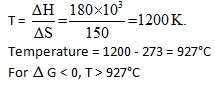Thermodynamics MCQ - 4 (Advanced) - Question 7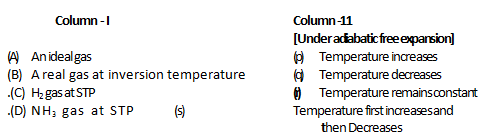Thermodynamics MCQ - 4 (Advanced) - Question 8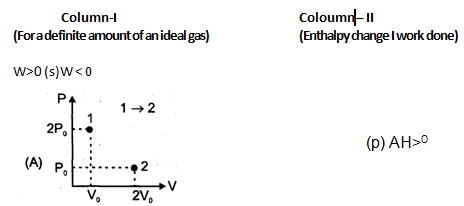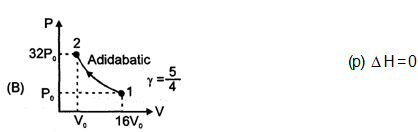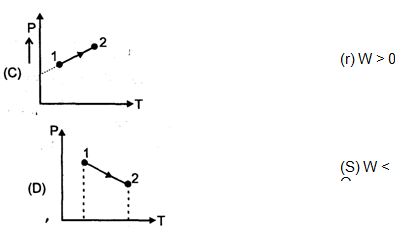Thermodynamics MCQ - 4 (Advanced) - Question 9

The feasibility of a chemical reaction can be explained based on H, S and G, so answer the following :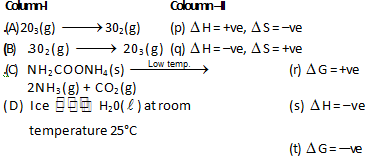Thermodynamics MCQ - 4 (Advanced) - Question 10

Match the following :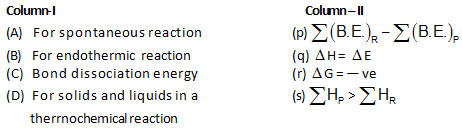Detailed Solution for Thermodynamics MCQ - 4 (Advanced) - Question 10

All the matching is obvious from the fact see the formula.

## Chemistry for JEE Advanced

12 videos|53 docs|118 tests
 Use Code STAYHOME200 and get INR 200 additional OFF Use Coupon Code
Information about Thermodynamics MCQ - 4 (Advanced) Page
In this test you can find the Exam questions for Thermodynamics MCQ - 4 (Advanced) solved & explained in the simplest way possible. Besides giving Questions and answers for Thermodynamics MCQ - 4 (Advanced), EduRev gives you an ample number of Online tests for practice

## Chemistry for JEE Advanced

12 videos|53 docs|118 tests